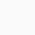# Robotics Foundations I – Robot Modeling

This course explores the robotics foundations for modelling of robot manipulators.

Robotics is commonly defined as the study of the intelligent connection between perception and action. As such, the full scope of robotics lies at the intersection of mechanics, electronics, signal processing, control engineering, computing, and mathematical modeling.

Within this very broad framework, modeling and control play a basic role – not only in the traditional context of industrial robotics, but also for the advanced applications of field and service robots, which have attracted increasing interest from the research community in the last twenty years.

Robotics foundations are dealt with in this two-part course. The first part covers robot modelling. Kinematics of robot manipulators is derived using a systematic approach based on the Denavit-Hartenberg convention and the use of homogeneous transformations.

The inverse kinematics problem is analyzed and closed-form solutions are found for typical manipulation structures. The Jacobian is then introduced as the fundamental tool to describe differential kinematics, determine singular configurations, analyze redundancy, derive the statics model and even formulate inverse kinematics algorithms.

The equations of motion of a robotic system are found on the basis of the dynamic model which is useful for motion simulation and control algorithm synthesis. Two approaches respectively based on Lagrange formulation and Newton-Euler formulation are pursued.

• The scenarios of industrial robotics and advanced robotics
• The fundamentals of kinematics, differential kinematics and statics
• The Jacobian and its properties
• The inverse kinematics algorithms
• The equations of motion of robot manipulators
Robotics Foundations I - Robot Modeling
-1

We will be happy to hear your thoughts